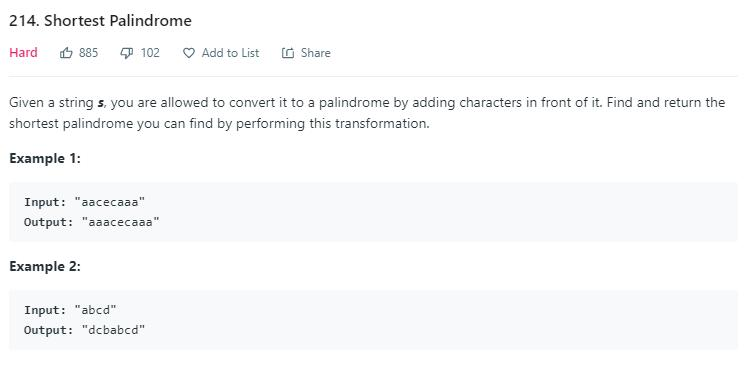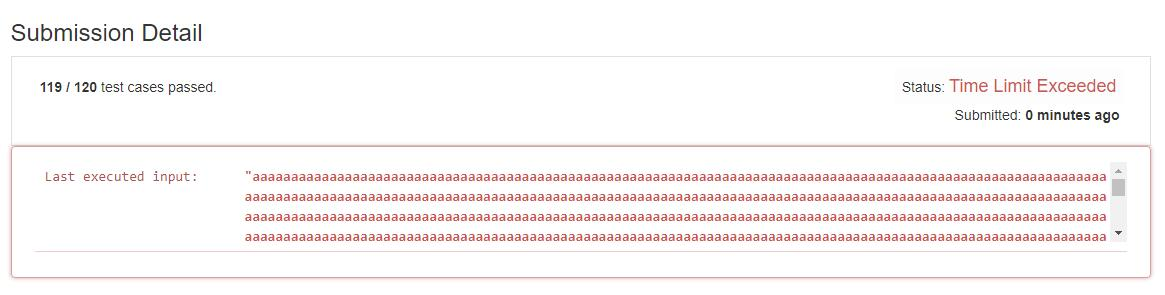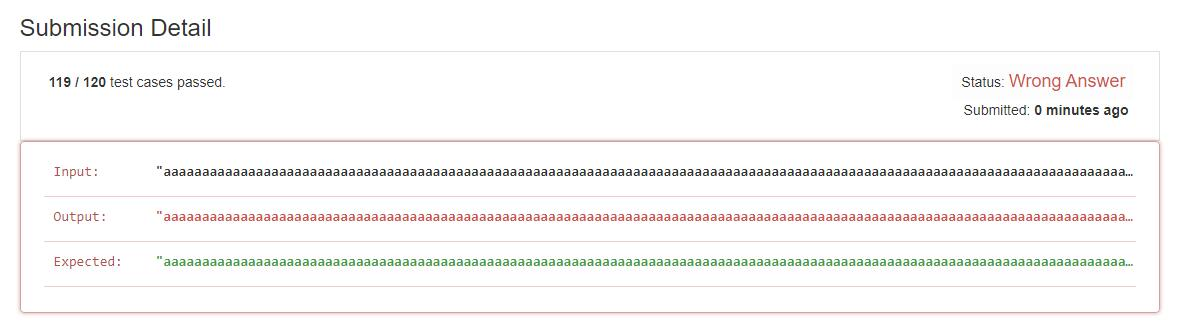# 题目描述（困难难度）# 解法一 暴力

...

原字符串 abbacd



//判断是否是回文串, 传入字符串的范围
public boolean isPalindromic(String s, int start, int end) {
char[] c = s.toCharArray();
while (start < end) {
if (c[start] != c[end]) {
return false;
}
start++;
end--;
}
return true;
}

public String shortestPalindrome(String s) {
int end = s.length() - 1;
//找到回文串的结尾, 用 end 标记
for (; end > 0; end--) {
if (isPalindromic(s, 0, end)) {
break;
}
}
//将末尾的几个倒置然后加到原字符串开头
return new StringBuilder(s.substring(end + 1)).reverse() + s;
}# 解法二

abbacde
a b b a c d e
^           ^
i           j

a b b a c d e
^         ^
i         j

a b b a c d e
^       ^
i       j

a b b a c d e
^     ^
i     j

a b b a c d e
^ ^
i j

a b b a c d e
^ ^
j i

a b b a c d e
^     ^
j     i

a b b a c d e
^        ^
j        i



abbacba
a b b a c b a
^           ^
i           j

a b b a c b a
^       ^
i       j

a b b a c b a
^   ^
i   j

a b b a c b a
^ ^
i j

a b b a c b a
^
i
j

a b b a c b a
^   ^
j   i

a b b a c b a
^     ^
j     i

a b b a c d e
^        ^
j        i



public String shortestPalindrome(String s) {
int i = 0, j = s.length() - 1;
char[] c = s.toCharArray();
while (j >= 0) {
if (i == j){
continue;
}
if (c[i] == c[j]) {
i++;
}
j--;
}
//此时代表整个字符串是回文串
if (i == s.length()) {
return s;
}
//后缀
String suffix = s.substring(i);
//后缀倒置
String reverse = new StringBuilder(suffix).reverse().toString();
//加到开头
return reverse + s;
}


ababbcefbbaba
a b a b b c e f b b a b a
^                       ^
i                       j

i 和 j 同时移动, 一直是相等, 直到下边的情况

a b a b b c e f b b a b a
^   ^
i   j

a b a b b c e f b b a b a
^            ^
j            i



public String shortestPalindrome(String s) {
int i = 0, j = s.length() - 1;
char[] c = s.toCharArray();
while (j >= 0) {
if (c[i] == c[j]) {
i++;
}
j--;
}
//此时代表整个字符串是回文串
if (i == s.length()) {
return s;
}
//后缀
String suffix = s.substring(i);
//后缀倒置
String reverse = new StringBuilder(suffix).reverse().toString();
//递归 s[0,i),寻找开头开始的最长回文串，将其余部分加到开头和结尾
return reverse + shortestPalindrome(s.substring(0, i)) + suffix;
}


aababababa
a a b a b a b a b a
^                 ^
i                 j

a a b a b a b a b a
^             ^
i             j

a a b a b a b a b a
^           ^
i           j

a a b a b a b a b a
^                ^
j                i

a a b a b a b a

a a b a b a

a a b a

a a



a a b a b a b a b a
a a b a b a b a
a a b a b a
a a b a
a a


# 解法三

abbacd

abbacd != dcabba

abbac != cabba

abba == abba



public String shortestPalindrome(String s) {
String r = new StringBuilder(s).reverse().toString();
int n = s.length();
int i = 0;
for (; i < n; i++) {
if (s.substring(0, n - i).equals(r.substring(i))) {
break;
}
}
return new StringBuilder(s.substring(n - i)).reverse() + s;
}


# 解法四

hash 算法，这里的话，我们将 a 看做 1b 看做 2 ... 以此类推，然后把字符串看做是 26 进制的一个数字，将其转为十进制后的值作为 hash 值。

 a      b    c    d
1      2    3    4
26^3  26^2   26   1


public String shortestPalindrome(String s) {
int n = s.length(), pos = -1;
int b = 26; // 基数
int pow = 1; // 为了方便计算倒置字符串的 hash 值
char[] c = s.toCharArray();
int hash1 = 0, hash2 = 0;
for (int i = 0; i < n; i++, pow = pow * b) {
hash1 = hash1 * b + (c[i] - 'a' + 1);
// 倒置字符串的 hash 值, 新增的字符要放到最高位
hash2 = hash2 + (c[i] - 'a' + 1) * pow;
if (hash1 == hash2) {
pos = i;
}
}
return new StringBuilder(s.substring(pos + 1)).reverse() + s;
}public boolean isPalindromic(String s, int start, int end) {
char[] c = s.toCharArray();
while (start < end) {
if (c[start] != c[end]) {
return false;
}
start++;
end--;
}
return true;
}
public String shortestPalindrome(String s) {
int n = s.length(), pos = -1;
int b = 26; // 基数
int pow = 1; // 为了方便计算倒置字符串的 hash 值
char[] c = s.toCharArray();
int hash1 = 0, hash2 = 0;
for (int i = 0; i < n; i++, pow = pow * b) {
hash1 = hash1 * b + (c[i] - 'a' + 1);
// 倒置字符串的 hash 值, 新增的字符要放到最高位
hash2 = hash2 + (c[i] - 'a' + 1) * pow;
if (hash1 == hash2) {
//确认下当前是否是回文串
if (isPalindromic(s,0,i)) {
pos = i;
}
}
}
return new StringBuilder(s.substring(pos + 1)).reverse() + s;
}public String shortestPalindrome(String s) {
int n = s.length(), pos = -1;
int b = 26; // 基数
int pow = 1; // 为了方便计算倒置字符串的 hash 值
char[] c = s.toCharArray();
int hash1 = 0, hash2 = 0;
int mod = 1000000;
for (int i = 0; i < n; i++, pow = (pow * b) % mod) {
hash1 = (hash1 * b + (c[i] - 'a' + 1)) % mod;
// 倒置字符串的 hash 值, 新增的字符要放到最高位
hash2 = (hash2 + (c[i] - 'a' + 1) * pow)% mod;
if (hash1 == hash2) {
pos = i;
}
}
return new StringBuilder(s.substring(pos + 1)).reverse() + s;
}


public String shortestPalindrome(String s) {
int n = s.length(), pos = -1;
int b = 26; // 基数
int pow = 1; // 为了方便计算倒置字符串的 hash 值
char[] c = s.toCharArray();
String rev = new StringBuilder(s).reverse().toString();
int hash1 = 0, hash2 = 0;
for (int i = 0; i < n; i++, pow = pow * b) {
hash1 = hash1 * b + (c[i] - 'a' + 1);
// 倒置字符串的 hash 值, 新增的字符要放到最高位
hash2 = hash2 + (c[i] - 'a' + 1) * pow;
if (hash1 == hash2) {
if (s.substring(0, i + 1).equals(rev.substring(n - i - 1))) {
pos = i;
}
}
}
return new StringBuilder(s.substring(pos + 1)).reverse() + s;
}


# 解法五

http://jakeboxer.com/blog/2009/12/13/the-knuth-morris-pratt-algorithm-in-my-own-words/

https://learnku.com/articles/10622/introduction-of-kmp-algorithm-and-derivation-of-next-array

abbacd

abbacd dcabba



public String shortestPalindrome(String s) {
String ss = s + '#' + new StringBuilder(s).reverse();
int max = getLastNext(ss);
return new StringBuilder(s.substring(max)).reverse() + s;
}

//返回 next 数组的最后一个值
public int getLastNext(String s) {
int n = s.length();
char[] c = s.toCharArray();
int[] next = new int[n + 1];
next = -1;
next = 0;
int k = 0;
int i = 2;
while (i <= n) {
if (k == -1 || c[i - 1] == c[k]) {
next[i] = k + 1;
k++;
i++;
} else {
k = next[k];
}
}
return next[n];
}


# 解法六

public String preProcess(String s) {
int n = s.length();
if (n == 0) {
return "^$"; } String ret = "^"; for (int i = 0; i < n; i++) ret += "#" + s.charAt(i); ret += "#$";
return ret;
}

// 马拉车算法
public String shortestPalindrome(String s) {
String T = preProcess(s);
int n = T.length();
int[] P = new int[n];
int C = 0, R = 0;
for (int i = 1; i < n - 1; i++) {
int i_mirror = 2 * C - i;
if (R > i) {
P[i] = Math.min(R - i, P[i_mirror]);// 防止超出 R
} else {
P[i] = 0;// 等于 R 的情况
}

// 碰到之前讲的三种情况时候，需要利用中心扩展法
while (T.charAt(i + 1 + P[i]) == T.charAt(i - 1 - P[i])) {
P[i]++;
}

// 判断是否需要更新 R
if (i + P[i] > R) {
C = i;
R = i + P[i];
}

}

//这里的话需要修改
int maxLen = 0;
int centerIndex = 0;
for (int i = 1; i < n - 1; i++) {
int start = (i - P[i]) / 2;
//我们要判断当前回文串是不是开头是不是从 0 开始的
if (start == 0) {
maxLen = P[i] > maxLen ? P[i] : maxLen;
}
}
return new StringBuilder(s.substring(maxLen)).reverse() + s;
}